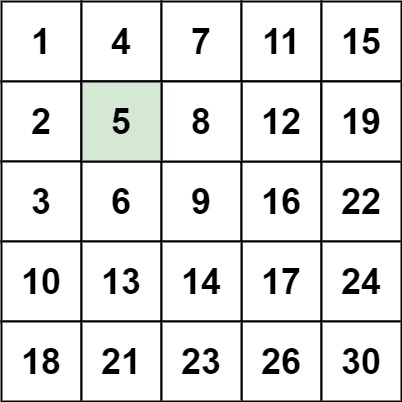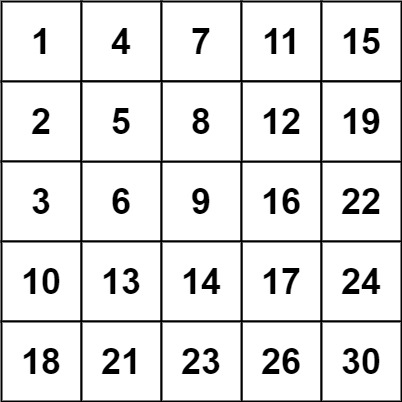# GeetCode Hub

Write an efficient algorithm that searches for a `target` value in an `m x n` integer `matrix`. The `matrix` has the following properties:

• Integers in each row are sorted in ascending from left to right.
• Integers in each column are sorted in ascending from top to bottom.

Example 1:```Input: matrix = [[1,4,7,11,15],[2,5,8,12,19],[3,6,9,16,22],[10,13,14,17,24],[18,21,23,26,30]], target = 5
Output: true
```

Example 2:```Input: matrix = [[1,4,7,11,15],[2,5,8,12,19],[3,6,9,16,22],[10,13,14,17,24],[18,21,23,26,30]], target = 20
Output: false
```

Constraints:

• `m == matrix.length`
• `n == matrix[i].length`
• `1 <= n, m <= 300`
• `-109 <= matix[i][j] <= 109`
• All the integers in each row are sorted in ascending order.
• All the integers in each column are sorted in ascending order.
• `-109 <= target <= 109`

class Solution { public boolean searchMatrix(int[][] matrix, int target) { } }